## Calculation of Efficiency from Performance Test Results

The principle behind calculation of efficiency is as follows:

• Losses 1a, 1b, and 2 are calculated from test results.

• Loss 3 is read from ABMA chart.

• Loss 4 is agreed before the test.

For calculating Lub (loss 2) in the case of PF-fired and FBC boilers, the ash distribution between fly ash and bottom or bed ash is often agreed upon, based on the boiler operation. This is because the loss is <2% and the sensitivity of error in the ash distribution is very small in comparison to the effort required to collect the data correctly. The code gives the following formulae for arriving at the losses 1a, 1b, and 2 using

• Flue gas analysis (CO2, CO, O2, N2 percent by volume in dry flue)

• Fuel analysis (C, H, S percent in fuel)

• Carbon in flue gas (Cg) and ash in percent by weight

• Temperature of exit gas and inlet air

• GCV or NCV in kilocalories per kilogram

The given formulae are used for calculating the losses 1a, 1b, and 2 on a GCV basis.

• Loss 1a:

 Dry gas loss (Ldg)%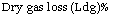(1.11)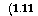W X 24(Tg — Ta) GCV

Where W is the weight of dry flue gas per kilogram of fuel.

 (1.12)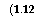W = 112 + 7(CO + N2) + 8O2 + _S_

3(CO2 + CO) ( g 183

Where Cg is the weight of carbon in flue gas per kilogram of fuel.

• Loss 1b:

 Moisture loss (Lm)%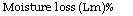(1.13)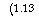[(100 — Ta) + 539 + 0.5 (Tg — 100)](9H2 + m)

GCV

TABLE 1.6

Measurements and Tolerances for Heat Loss Method

 Measurement Measurement Error (%) Error in Calculation of Boiler Efficiency (%) Gross calorific value 0.50 0.03 (coal) Gross calorific value 0.35 0.02 (oil/gas) Orsat analysis 3.00 0.30 Exit gas temperature 0.50 0.02 Inlet air temperature 0.50 0.00 Ultimate analysis of 1.00 0.10 Coal—C, H2 H2O in fuel 1.00 0.00

 Note: All figures in percentage are ± for both error and efficiency; air and gas temperature measurements were made by calibrated devices.

• Loss 2:

TT, , /T, … percent ash in fuel X Ca X 80.78

Unburnt loss (Lub)% = ——————— ————- a————

Where Ca is percentage of combustibles in fly and bottom or bed ash.

PTC 4.1 provides for a certain tolerance when it specifies measurement errors for all types of instruments used in testing and the corresponding error in calculated efficiency. This works out to approximately ±0.3% for the overall efficiency figure.

Table 1.6 lists the accepted measurement error of each variable and its effect on the test result. The maximum effects are, as shown in the table, in the analyses of coal by bomb calorimeter and gas by Orsat analyzer.

Комментирование и размещение ссылок запрещено.

Комментарии закрыты.

gazogenerator.com